Physics
Fox

### Summary

• Below is a recap of what we've learnt about circuits, and some extra questions to help you practice.

Electrical circuits are one of the most challenging things at this stage of physics, so it's important to make sure you understand them!

Here is a summary of everything we've learnt about circuits (so far):

Everything is made from atoms. Atoms are themselves made from protons, neutrons and electrons. Electrons have a charge, which means they can be pushed around with the electric force. Electrons are free to move in materials called conductors (but cannot flow in insulators).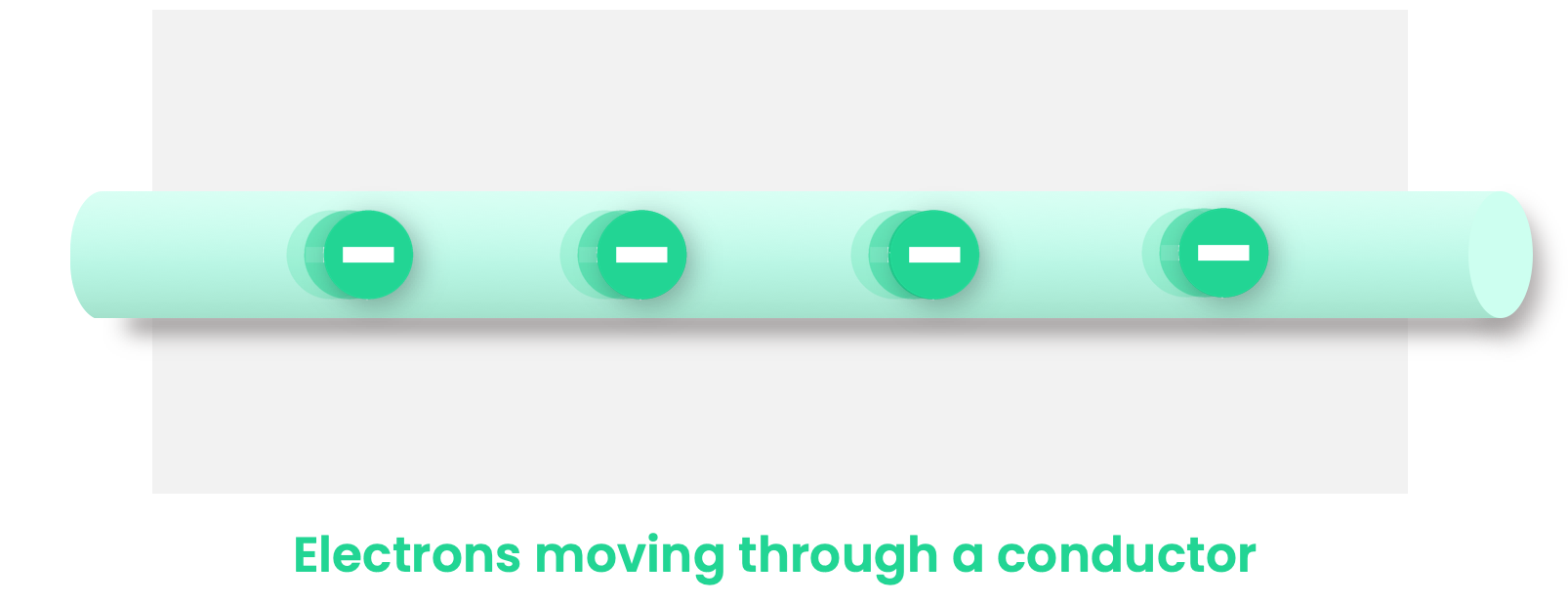Circuits are made from paths of conductors (often copper wires). Cells and batteries provide the electric force to push the electrons around the circuit. A series circuit goes along a single path, and a parallel circuit goes along multiple paths.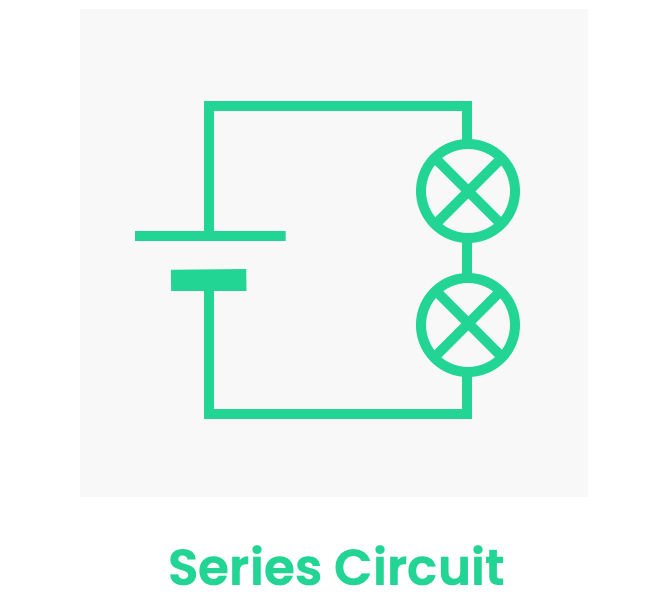The two most important quantities used for understanding electricity are current and potential difference:

Current is the rate of flow of electrons. Conventional current goes from the + to - terminal of a cell or battery, if there is a path it can take (a complete circuit). Current is measured in units of Amps. Current is always the same in a series circuit, but splits in a parallel circuit. Current is measured using an ammeter (which must be placed in series).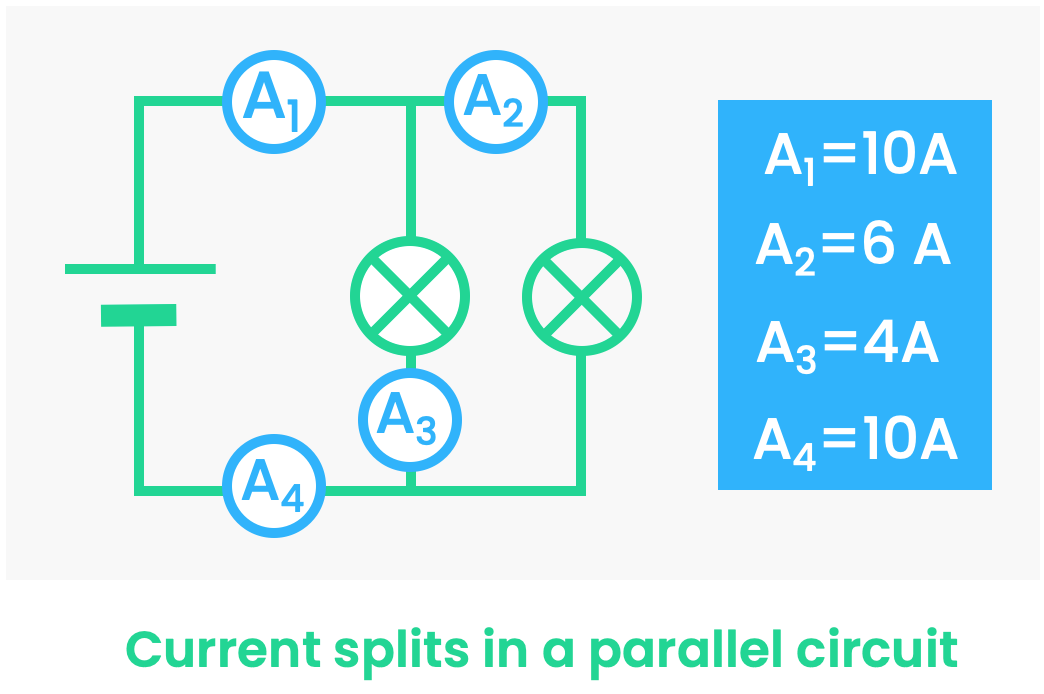Potential difference (or voltage) is the difference in potential energy for each electron. It is measured in units of Volts. Potential difference is split between components in a series circuit, does not split in parallel circuit. Voltage is measured using an voltmeter (which must be placed in parallel).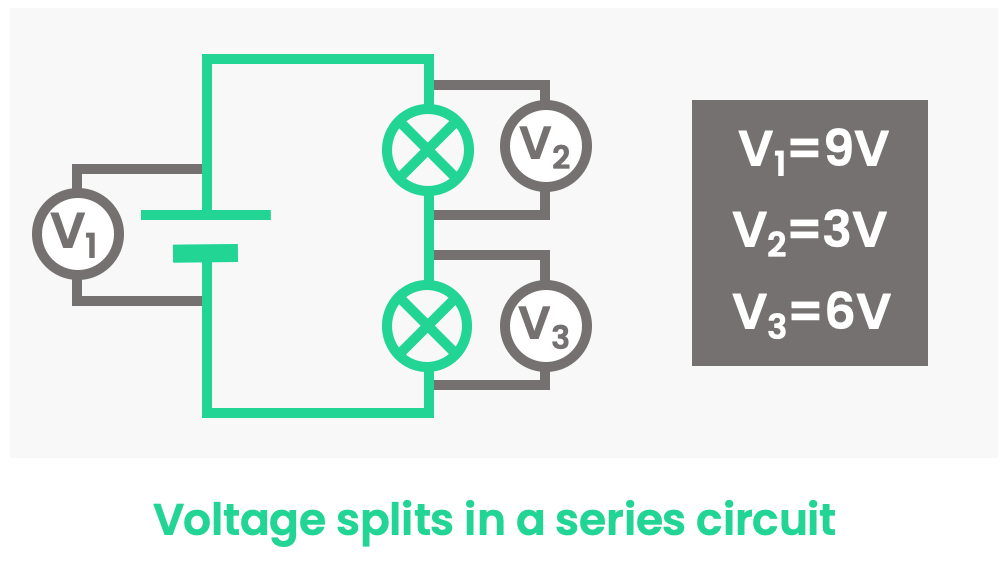Current cannot flow through a closed switch, but it can flow through an open switch.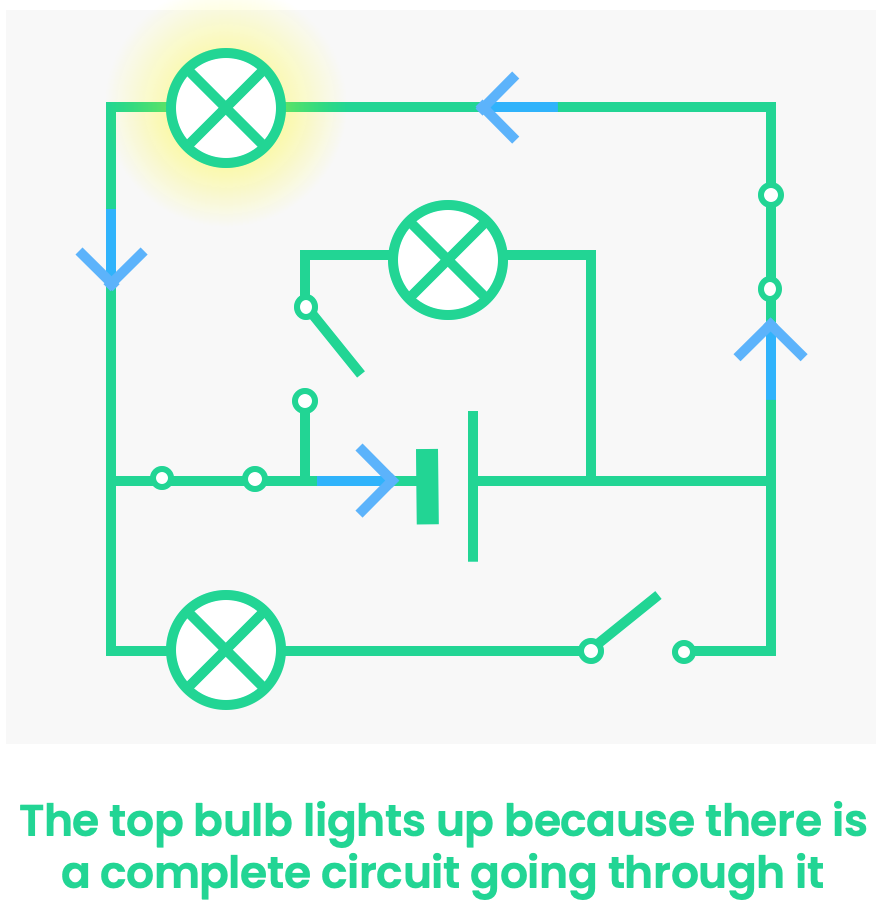Quiz

### Congratulations!

32 of 32 questions completed

+ ⭐️ collected.

• You can change your display name at any time.
• at least 1 letter
• at least 1 number
• at least 8 characters